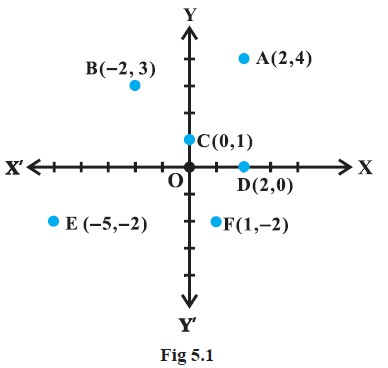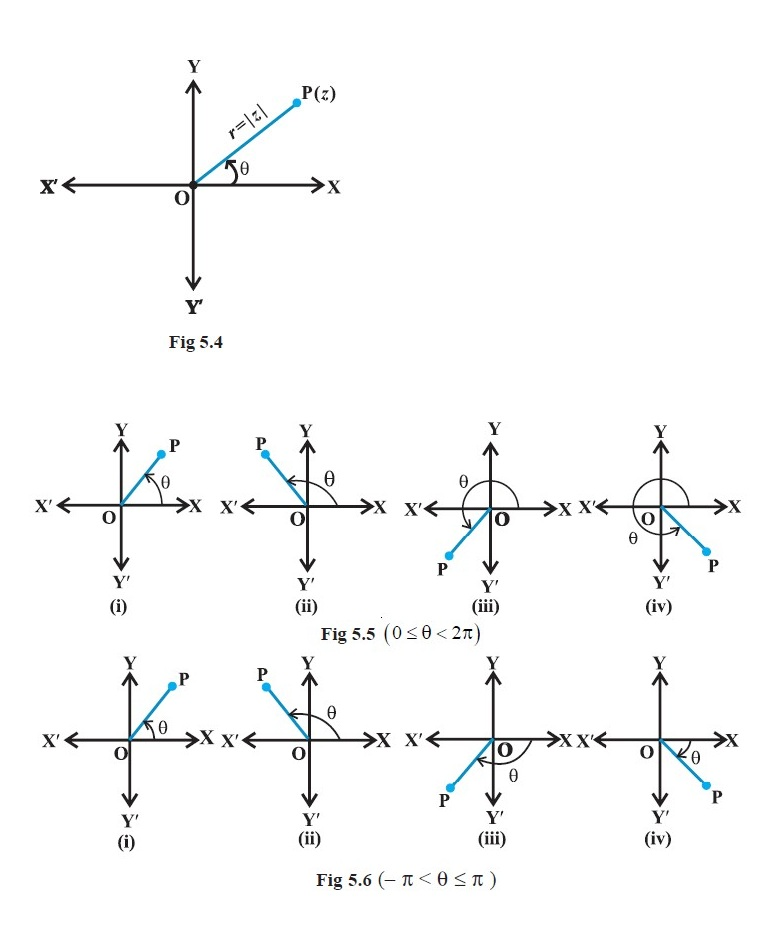Mathematics COMPLEX NUMBERS- Argand Plane and Polar Representation
Click for Only Video

### Topics Covered

star Argand Plane
star Modulus of the complex number in the Argand plane
star Representation of a complex number and its conjugate
star Polar representation of a complex number

### Argand Plane\color{green} ✍️  The plane having a complex number assigned to each of its point is called the color(blue)"complex plane or the Argand plane."

\color{green} ✍️  We already know that corresponding to each ordered pair of real numbers (x, y), we get a unique point in the XY plane and vice-versa with reference to a set of mutually perpendicular lines known as the x-axis and the y-axis.

\color{green} ✍️  The complex number x + iy which corresponds to the ordered pair (x, y) can be represented geometrically as the unique point P(x, y) in the XY-plane and vice-versa.

\color{green} ✍️  Some complex numbers such as 2 + 4i, – 2 + 3i, 0 + 1i, 2 + 0i, – 5 –2i and 1 – 2i which correspond to the ordered pairs (2, 4), ( – 2, 3), (0, 1), (2, 0), ( –5, –2), and (1, – 2), respectively, have been represented geometrically by the points A, B, C, D, E, and F, respectively in the Fig 5.1.

\color{green} ✍️  The points on the x-axis corresponds to the complex numbers of the form a + i 0
and the points on the y-axis corresponds to the complex numbers of the form 0 + i b.

\color{green} ✍️  The x-axis and y-axis in the Argand plane are called, respectively, the color(blue)"real axis" and the color(blue)"imaginary axis."

### modulus of the complex number in the Argand plane\color{green} ✍️  color(blue)(|x + iy| = sqrt(x^2 + y^2)) is the distance between the point P(x, y) to the origin O (0, 0) (Fig 5.2).

### representation of a complex number and its conjugate\color{green} ✍️  The representation of a complex number color(blue)(z = x + iy) and

its conjugate color(blue)(z = x – iy) in the Argand plane are, respectively, the points color(blue)(P (x, y)) and color(blue)(Q (x, – y)).

\color{green} ✍️ Geometrically,
color(blue)("the point (x, – y) is the mirror image of the point (x, y) on the real axis") (Fig 5.3).

### Polar representation of a complex number\color{green} ✍️ Let the point P represent the nonzero complex number color(blue)(z = x + iy.)

\color{green} ✍️ Let the directed line segment OP be of length r and θ be the angle which OP makes with the positive direction of x-axis (Fig 5.4).

\color{green} ✍️ We may note that the point P is uniquely determined by the ordered pair of real numbers color(blue)((r, θ)), color(red)("called the polar coordinates of the point P.") We consider the origin as the pole and the positive direction of the x axis as the initial line.

\color{green} ✍️ We have, color(blue)(x = r cos θ, y = r sin θ) and therefore, color(blue)(z = r (cos θ + i sin θ).) The latter is color(red)("said to be the polar form of the complex number. ")

\color{green} ✍️ Here color(blue)(r = sqrt(x^2 + y^2) = |z|) is the modulus of z and θ is called the argument (or amplitude) of z which is denoted by arg z.

\color{green} ✍️ For any complex number z ≠ 0, there corresponds only one value of θ in 0 ≤ θ < 2π. However, any other interval of length 2π, for example – π < θ ≤ π, can be such an interval.

\color{green} ✍️ We shall take the value of θ such that color(red)(– π < θ ≤ π), called color(red)("principal argument of z") and is denoted by arg z . (Figs. 5.5 and 5.6)
Q 3018712609Represent the complex number z = 1+i sqrt3 in the polar form.Solution:

Let 1 = r cos θ, sqrt3 = r sintheta

By squaring and adding, we get

r^2 (cos^2 theta+sin^2 theta) = 4

i.e. r = sqrt3 = (conventionally, r > 0)

Therefore cos theta = 1/2 , sintheta = sqrt3/2 which gives theta = pi/3

Therefore, required polar form is z = 2 ( cos \ \ pi/3 + i sin \ \ pi/3)

The complex number z = 1+i sqrt3 is represented as shown in Fig
Q 3088012807Convert the complex number (-16)/(1+i sqrt3) into polar form.Solution:

The given complex number (-16)/(1+i sqrt3) = (-16)/(1+i sqrt3) xx (1- i sqrt3)/(1-i sqrt3)

 = (-16 (1-i sqrt3))/{1- (i sqrt3)^2} = { -16 (1-i sqrt3)}/(1+3) = -4(-i sqrt3) = -4+i sqrt3

Let -4 = rcostheta , sqrt3 = rsintheta

By squaring and adding, we get

16+48 = r^2 ( cos^2 theta +sin^2 theta)

where gives r^2 = 64 , i. e , r = 8

Hence costheta = -1/2 , sintheta = sqrt3/8

theta = pi- pi/3 = (2pi)/3

Thus, the required polar form is 8 ( cos \ \ (2pi)/3 + i sin \ \ (2pi)/3)